Technical Article

# PLC Program Commands: Performing Array Functions Part 2

November 22, 2022 by Jon Peterson

## Learn about multi-dimensional arrays and how to work with them to perform math operations such as the AVE (Average) instruction in the Rockwell (Allen-Bradley) Studio 5000 programming software.

#### See our previous article on Array Functions:

PLC Program Commands: Performing Array Functions Part 1

### Understanding Multi-dimensional Arrays

Nearly every day that I work with PLCs, I use arrays in some form or another. Arrays are used to group large amounts of similar data into one cohesive tag format. I always try to make real-world examples, so let’s discuss a basic array first

#### 1D Array in a PLC

I want to store the number of pieces a machine has made per hour at the end of each hour. To accomplish this, I write a series of instructions that adds a new element of data to the array every hour. When I’m done, this is what the first 6 hours might look like below.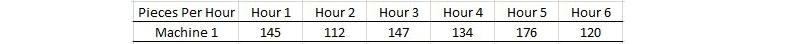##### Figure 1. A 1D array displaying pieces produced each hour.

This is a single-dimension array of data. Position one has a single number for a single machine. Position two has a single number for the same machine. Every time you add an hour, you add a single position to the list.

#### 2D Array in a PLC

Now we need to add the other three machines in our facility to the array to get their piece counts every hour. Here’s what we get when we do that. We now are recording data from 4 identical machines in an organized fashion, every hour.##### Figure 2. This 2D array still shows parts per hour, but now the second dimension stores data from multiple machines at once.

You may have heard of two-dimensional arrays before. Maybe you thought they were far too complicated to work with in PLCs. The example above is a two-dimensional array! The first hour has four piece counts, the second hour has four piece counts, and on, and on.

I wish it was just as simple to take this idea and apply it directly from Excel to Studio 5000, but it’s a bit more difficult. Let’s take a look at how Rockwell’s (Allen-Bradley’s) Studio 5000 software shows an array with two dimensions.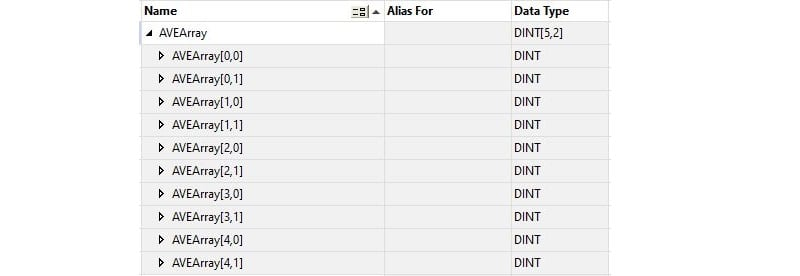##### Figure 3. A single 2D array with 5 rows and 2 columns in Studio 5000. Note the array index with two numbers (e.g. [0,0]) to indicate the row and column.

You’ll notice that the array is still displayed in a single column. This is just a trick of the Tag Database layout. The important information is in the tag brackets. Element 0,0 in our array would be row 1, position 1 in our Excel layout. Element 0,1 would be row 1, position 2 in Excel - Machine 2’s piece count. We then move on to Element 1,0 which would be Machine 1, Hour 2.

Now, why do we care? This is always the question isn’t it? The reason we are covering this is because we are about to address some instructions that can be used on specific dimensions of our arrays. For example, the AVERAGE instruction can average the ‘0’ dimension of the array or the ‘1’ dimension. To translate this back to our example, we could average Machine 1 over six hours (the 1st row), or we could average all the machines’ piece counts for a specific hour (the 1st column).

The array we created in Studio 5000 above has five columns and two rows. The rows are noted in the first number before the comma and the columns are the second number, after the comma.

#### 3D Array in a PLC

There’s one last thing to introduce you to: the three-dimensional array. I will show this to you to make you aware of its existence. If you want to study it further, that’s great, but think about it like this: a one-dimensional array is a line, a two-dimensional array is a sheet (or flat plane), and a three-dimensional array is a cube. There are many more dimensions in mathematical arrays, but beyond three dimensions, they are very hard to visualize in tangible, logical terms for us humans.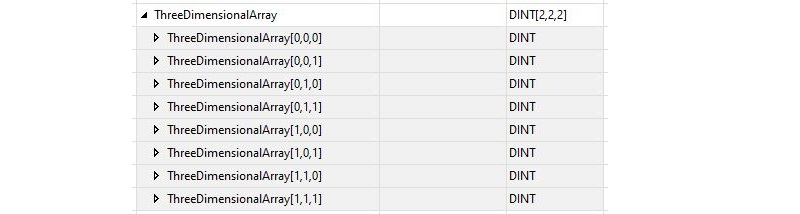##### Figure 4. Three dimensions in one array, the naming notion now becoming ‘length, width, and height’ since ‘row and column’ fail to provide a third dimension in most spreadsheet applications.

Isn’t it awesome, that much data in one single tag? Well, I think it is! But now, let’s move on to the instructions we can use to work with it.

### Using the AVE (File Average) Instruction in Studio 5000

I think there’s no better place to start than with the averaging function we described above.

The first step we take in understanding instructions is to define the tags used to make the instruction work. The AVE instruction begins by defining the array that we are going to be averaging. This array can be any conventional INT or REAL datatype and can be any number of dimensions. We always plug in our array by defining the name and the first element in that array. In the logic you see below, the first element in our AVEArray is [0,0], the first element of a two-dimensional array.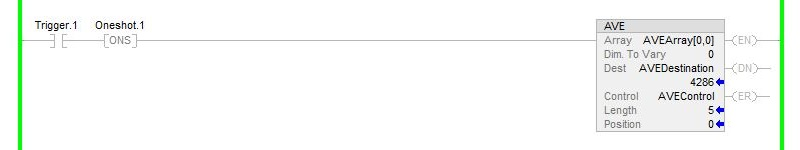##### Figure 5. Average (AVE) instruction operating on only the first 5 instances of the 0th (the first) dimension of an array.

The next thing we have to define is the dimension of the array that we want to work with. Remember, the first number is a Column and the second number is a Row. I am choosing to average the first column of data in my array.

Now we move on to where we want to store the data that we get as a result of our average. I called my AVEDestination for clarity’s sake. Remember that if you need to be really accurate, you may need to use a REAL datatype when averaging DINTs in an array. The average could still have a decimal value.

Next up is our Control tag. For more info on exactly what the Control tag does, consult our previous article. The length needs to be set to the number of items in our array. Now be careful here! If you have a two-dimensional array, like I do above, your first dimension could be five elements long while the second dimension is only two elements long. If you are averaging the second dimension, you want the length to match the number of values in that dimension only.

Now let’s take a closer look at how my instruction averaged the data. In the AVE command, I averaged the first dimension (Dimension ‘0’) and only the first 5 array elements, and my result was 4286. Here’s the data I started with.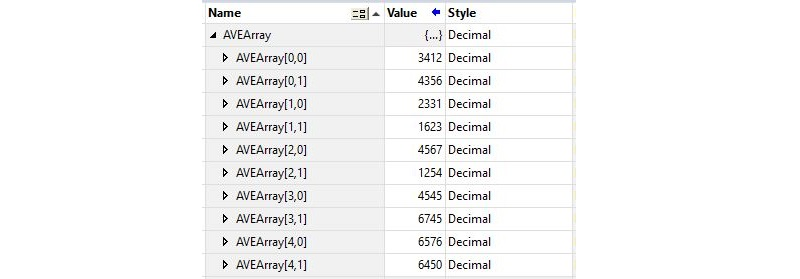##### Figure 6. The original array that was used to calculate the average in the previous Figure (Fig. 5). Note that the result of the averaging calculation in the previous Figure was 4286.

Our instruction averaged the numbers in the first dimension—the first number in the brackets. But did it actually do this? Here’s what it looks like in math syntax, let’s verify it.

(3412 + 2331 + 4567 + 4545 + 6576)/5 = 4286.2

That matches up with our result! The first dimension was properly averaged. Now remember back to what I mentioned about averaging DINTs and coming up with a REAL? This is a perfect example of it. I ended up with a decimal point in my calculator, but because I placed the value in a DINT, it had to drop the decimal place. When you place a REAL number into a DINT, it doesn’t round up or down, it simply drops the decimal places. If you put 2.99 into a DINT, you’d get 2. This could create havoc in a finely tuned system.

I hope you enjoyed the foray into the world of multi-dimensional arrays as much as I enjoyed writing it!

Want to test your knowledge of PLCs? You think you N.O. a lot about ladder logic? Are you a normally-open or normally-closed minded engineer?

Check out our PLC Programming worksheet!

##### Featured image used courtesy of Keyence